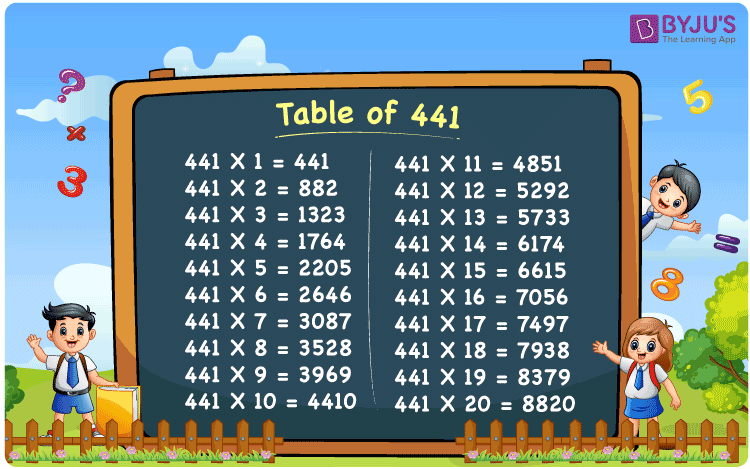Checkout JEE MAINS 2022 Question Paper Analysis : Checkout JEE MAINS 2022 Question Paper Analysis :

# Table of 441

The Table of 441 represents the multiples produced by the multiplication of 441 with the consecutive natural numbers. 441 times table can also be generated by the repeated addition method. Learn the table of 441 here in this article and solve multiplication related problems quickly. Find more about Multiplication tables at BYJU’S and download the PDF of table 441 here for free.

## Table of 441 Chart## What is 441 Times Table?

The table below shows the repeated addition of the number 441 to get the required multiple.

 441×1 = 441 441 441×2 = 882 441 + 441 = 882 441×3 = 1323 441 + 441 + 441 = 1323 441×4 = 1764 441 + 441 + 441 + 441 = 1764 441×5 = 2205 441 + 441 + 441 + 441 + 441 = 2205 441×6 = 2646 441 + 441 + 441 + 441 + 441 + 441 = 2646 441×7 = 3087 441 + 441 + 441 + 441 + 441 + 441 + 441 = 3087 441×8 = 3528 441 + 441 + 441 + 441 + 441 + 441 + 441 + 441 = 3528 441×9 = 3969 441 + 441 + 441 + 441 + 441 + 441 + 441 + 441 + 441 = 3969 441×10 = 4410 441 + 441 + 441 + 441 + 441 + 441 + 441 + 441 + 441 + 441 = 4410

## Multiplication Table of 441

Here is the table of 441 up to 20 times below.

 441 × 1 = 441 441 × 2 = 882 441 × 3 = 1323 441 × 4 = 1764 441 × 5 = 2205 441 × 6 = 2646 441 × 7 = 3087 441 × 8 = 3528 441 × 9 = 3969 441 × 10 = 4410 441 × 11 = 4851 441 × 12 = 5292 441 × 13 = 5733 441 × 14 = 6174 441 × 15 = 6615 441 × 16 = 7056 441 × 17 = 7497 441 × 18 = 7938 441 × 19 = 8379 441 × 20 = 8820

## Solved Example on Table of 441

Find the value of (441 x 3) – 956

Solution: Given,

441 x 3 – 956

From the table of 441, we have;

441 x 3 = 1323

Therefore,

1323 – 956 = 367

## Frequently Asked Questions on Table of 441

### What is table of 441 in Maths?

The table of 441 is the multiplication table that expresses the multiples of the actual number, i.e., 441.

### What is the value of 441 x 11?

The value of 441 times 11 is equal to 4851.

### How many times should we add 441 to get 2646?

441 should be added to itself six times to get 2646, since 441 x 6 = 2646.